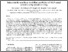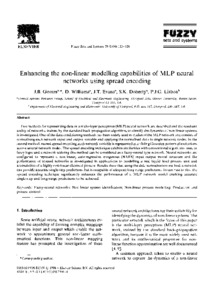# Enhancing the non-linear modelling capabilities of MLP neural networks using spread encoding

Gomm, J.B. and Williams, D. and Evans, J.T. and Doherty, Sean and Lisboa, P.J.G. (1996) Enhancing the non-linear modelling capabilities of MLP neural networks using spread encoding. Fuzzy Sets and Systems, 79 (1). pp. 113-126. ISSN 0165-0114Previewmore...Add this article to your Mendeley library

## Abstract

Two methods for representing data in a multi-layer perceptron (MLP) neural network are described and the resultant ability of networks, trained by the standard back-propagation algorithm, to identify the dynamics of non-linear systems is investigated. One of the data conditioning methods has been widely used in studies of the MLP network and consists of normalising each network input and output variable and applying the normalised data to single network nodes. In the second method, named spread encoding, each network variable is represented as a sliding Gaussian pattern of excitations across several network nodes. The spread encoding technique exhibits similarities with conventional algorithms used in fuzzy logic and a network utilising this method can be considered as a fuzzy-neural type network. Neural networks are configured to represent a non-linear, auto-regressive, exogenous (NARX) input-output model structure and the performance of trained networks is investigated in applications to modelling a real liquid level process unit and a simulation of a highly non-linear chemical process. Results show that using the data normalisation method, a network can provide accurate single-step predictions but is incapable of adequate long-range predictions. In contrast to this, the spread encoding technique significantly enhances the performance of a MLP network model enabling accurate single-step and long-range predictions to be achieved.

Item Type: Article Fuzzy-neural networks; Non-linear system identification; Non-linear process modelling; Productionprocess control; Faculty of Science and Engineering > Electronic Engineering 9684 https://doi.org/10.1016/0165-0114(95)00294-4 Seán Doherty 17 Jul 2018 15:48 Fuzzy Sets and Systems Elsevier YesItem control page